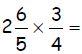Name:    Math 9 Final Exam review #1

Multiple Choice
Identify the choice that best completes the statement or answers the question.

1.

The following is a rational number?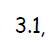a. TRUE b. FALSE

2.

Which of the following is not a rational number?
 a.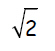c.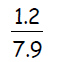b.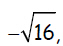d.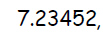3.

Order the following rational numbers from least to greatest.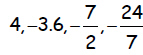a.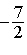,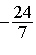, -3.6, 4 c., -3.6,, 4 b. -3.6,,, 4 d. -3.6,,, 4

4.

Converting rational numbers to the aame form, (all fractions or all decimals), is often a good when you are trying to compare them.
 a. True b. False

5.

Evaluate: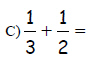a.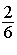c.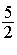b.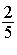d.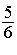6.

Evaluate the following: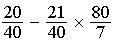a.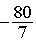c. -5.5 b.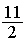d. 6.5

7.

Evaluate the expression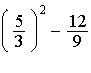a. 0 c.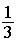b.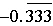d.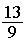8.

The following formula converts degrees Fahrenheit (F) to degrees Celsius (C).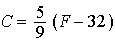.  Convert 59 degrees Fahrenheit to degrees celsius.
 a. 15 c. 13 b. 14 d. 12

Multiple Response
Identify one or more choices that best complete the statement or answer the question.

9.

Given the following list of numbers, check all the ones that are rational numbers.  There could be more than one answer:
 a.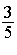d.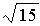b.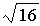e.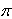c.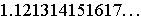f.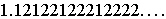10.

Which of the following are rational numbers.  Check all that apply.  There could be more than one answer.
 a. 0.3333... c.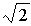b. -1.252525.... d. 7.23452

Matching

Match the letter to the appropriate number:
 a. Variable f. Trinomial b. Term g. Polynomial c. Binomial h. Coefficient d. Monomial i. Degree e. Constant

11.

What is the “x” called in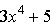12.

What is the “3” called in13.

What is the “5” called in14.

What is the “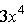” called in15.

What does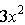,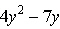, and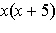all have in common?

16.

2y is an example of what?

17.is an example of what?

18.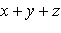is an example of what?

19.andare both exaples of what?

Problem

20.